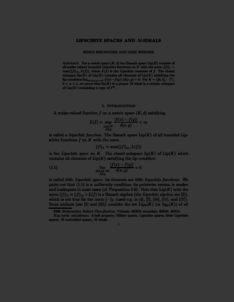Repository: Freie Universität Berlin, Math Department

# Lipschitz spaces and M-ideals

Berninger, H. and Werner, Dirk (2003) Lipschitz spaces and M-ideals. Extracta Mathematicae, 18 (1). pp. 33-56. ISSN 0213-8743Preview

341kB

Official URL: http://www.eweb.unex.es/eweb/extracta/

## Abstract

For a metric space (K,d) the Banach space Lip(K) consists of all scalar-valued bounded Lipschitz functions on K with the norm ||f||_L = max(||f||_{\infty}, L(f)), where L(f) is the Lipschitz constant of f. The closed subspace lip(K) of Lip(K) contains all elements of Lip(K) satisfying the lip-condition lim_{0 < d(x,y) -> 0} |f(x)-f(y)|/d(x,y) = 0. For K=([0,1], |.|^{alpha}), 0 < alpha < 1, we prove that lip(K) is a proper M-ideal in a certain subspace of Lip(K) containing a copy of l^{\infty}.

Item Type: Article Mathematical and Computer Sciences > Mathematics > Numerical Analysis Department of Mathematics and Computer Science > Institute of Mathematics 1833 Ekaterina Engel 06 Mar 2016 12:33 03 Mar 2017 14:42

Repository Staff Only: item control page